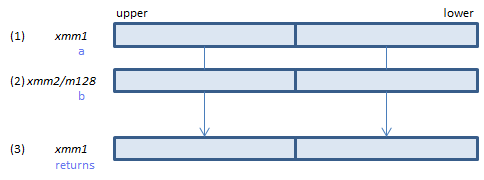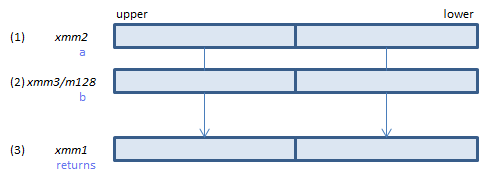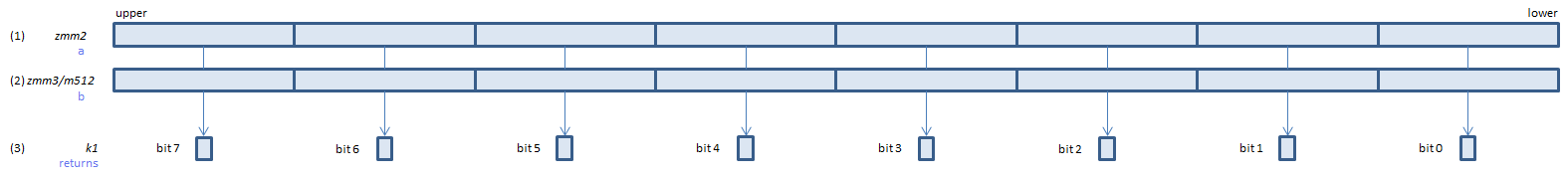﻿ cmplepd

## CMPLEPD - CoMPare Less Equal Packed Double

CMPLEPD xmm1, xmm2/m128    (S2
__m128d _mm_cmple_pd(__m128d a, __m128d b)For each double, if (1) <= (2) set 1, else set 0, to all bits of the corresponding double of (3).
VCMPLEPD xmm1, xmm2, xmm3/m128    (V1
__m128d _mm_cmple_pd(__m128d a, __m128d b)For each double, if (1) <= (2) set 1, else set 0, to all bits of the corresponding double of (3).
VCMPLEPD ymm1, ymm2, ymm3/m256    (V1
__m256d _mm256_cmp_pd(__m256d a, __m256d b, _CMP_LE_OS)For each double, if (1) <= (2) set 1, else set 0, to all bits of the corresponding double of (3).
VCMPLEPD k1{k2}, xmm2, xmm3/m128/m64bcst    (V5+VLFor each double, if (1) <= (2) set 1, else set 0, to the corresponding bit of (3).
If k2 bit is 0, the comparison is not done and the corresponding bit of (3) is set to zero. Upper bits of (3) are zero cleared.
VCMPLEPD k1{k2}, ymm2, ymm3/m256/m64bcst    (V5+VLFor each double, if (1) <= (2) set 1, else set 0, to the corresponding bit of (3).
If k2 bit is 0, the comparison is not done and the corresponding bit of (3) is set to zero. Upper bits of (3) are zero cleared.
VCMPLEPD k1{k2}, zmm2, zmm3/m512/m64bcst{sae}    (V5